# What Is The Focus Of A Parabola? (3 Things To Remember)

For the graph of a parabola, we would use a quadratic equation y = ax2 + bx + c to represent it.  However, there is another way: using a point (the focus) and a horizontal line (the directrix).

So, what is the focus of a parabola?  The focus of a parabola is a point that helps to define the graph, along with a horizontal line (called the directrix). The focus is found on the parabola’s axis of symmetry (the same vertical line that goes through the vertex).  The vertex is always halfway between the focus and the directrix.

Of course, we can find the focus and the directrix from the standard equation of a parabola (quadratic).

In this article, we’ll talk about the focus of a parabola and how it relates to the directrix.  We’ll also take a look at some examples of ways to find the focus of a parabola.

Let’s get started.

## What Is The Focus Of A Parabola?

The focus of a parabola is a point that helps us to figure out what the graph looks like.  However, the focus alone is not enough.

We also need to use the directrix, which is a horizontal line that does not intersect the parabola.  You can see the graph of a convex parabola below (opens upward), which also illustrates the focus and the directrix.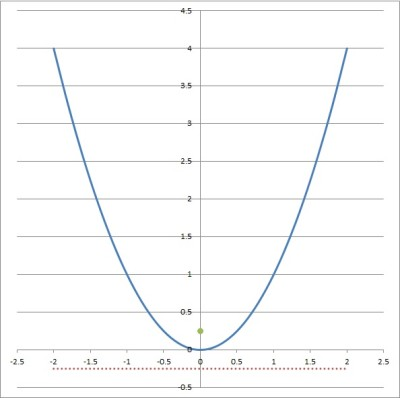Here, we have the parabola y = x2 (blue curve), along with the focus at the green point (0, 1/4) and the directrix y = -1/4 (horizontal red line).

Looking at the graph, we can see that the focus and vertex are on the same vertical line (the axis of symmetry).

Also note that the vertex (the lowest point of a convex parabola) is between the focus and the directrix (below the focus and above the directrix).

For a concave parabola (opens downward), the vertex would still be between the focus and directrix, but it would be above the focus and below the directrix.

Now that we know what the focus of a parabola is, let’s look at some of the ways to find it.

## How Do You Find The Focus Of A Parabola?

For any parabola, if the vertex is the point (h, k), then the focus is (h, k + (1/4a)), where a is the quadratic coefficient of y = ax2 + bx + c.

Since the vertex and focus are on the same vertical line (the axis of symmetry), it makes sense that the x-coordinate would be the same for both (it is h).

This also tells us that the focus is a distance of 1/4|a| from the vertex.

So, no matter which form of a parabola we have, we will be able to find the focus if we find the vertex (h, k) and the quadratic coefficient a.

*Note: this also means that the directrix has the equation y = k – (1/4a).

### How To Find The Focus Of A Parabola In Vertex Form

A parabola in vertex form has the equation:

• y = a(x – h)2 + k

where a, h, and k are real numbers and a is nonzero.

The vertex of the parabola is the point (h, k), which we can easily read from the equation (thus its name, the vertex form).

We can also easily find a from the vertex form, which gives us a, h, and k – everything we need to find the focus.

Let’s take a look at some examples.

#### Example 1: Find The Focus Of A Parabola In Vertex Form

Let’s say we have the following quadratic equation in vertex form:

• y = 2(x – 5)2 + 7

In this case, a = 2, h = 5 and k = 7.  This means that the vertex of the corresponding parabola is (h, k) = (5, 7).

We can verify this with the graph below.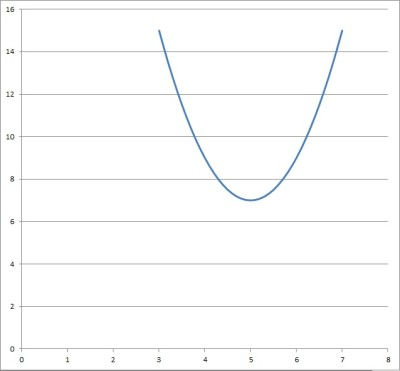Here, we have the parabola y = 2(x-5)2 +7, with a vertex at the point (5, 7).

According to our equation above, the focus is the point (h, k + 1/4a).

Substituting a = 2, h = 5, and k = 7, we get:

• an x-coordinate of h = 5
• a y-coordinate of k + 1/4a = 7 + 1/4(2) = 7 + 1/8 = 7.125

So, the focus of this parabola is the point (5, 7.125).

Likewise, the directrix is the line y = k – (1/4a), which is y = 6.875 when a = 2.

You can see the focus and directrix illustrated on the graph below.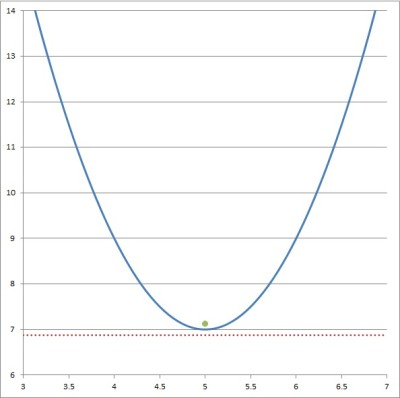Here, we have the parabola y = 2(x-5)2 +7 (blue curve), along with the focus at the green point (5, 7.125) and the directrix y = 6.875 (horizontal red line).

#### Example 2: Find The Focus Of A Parabola In Vertex Form

Let’s say we have the following quadratic equation in vertex form:

• y = 5(x – 3)2 + 4

In this case, a = 5, h = 3 and k = 4.  This means that the vertex of the corresponding parabola is (h, k) = (3, 4).

We can verify this with the graph below.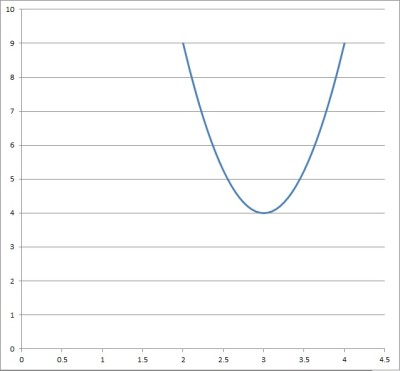Here, we have the parabola y = 5(x-3)2 +4, with a vertex at the point (3, 4).

According to our equation above, the focus is the point (h, k + 1/4a).

Substituting a = 5, h = 3, and k = 4, we get:

• an x-coordinate of h = 3
• a y-coordinate of k + 1/4a = 4 + 1/4(5) = 4 + 1/20 = 4.05

So, the focus of this parabola is the point (3, 4.05).

Likewise, the directrix is the line y = k – (1/4a), which is y = 3.95 when a = 5.

You can see the focus and directrix illustrated on the graph below.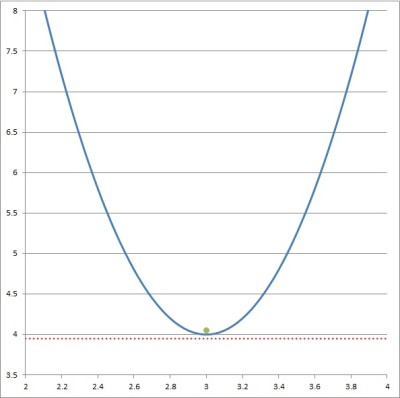Here, we have the parabola y = 5(x-3)2 +4 (blue curve), along with the focus at the green point (3, 4.05) and the directrix y = 3.95 (horizontal red line).

### How To Find The Focus Of A Parabola In Standard Form

One way to find the focus of a parabola in standard form is to convert to vertex form and follow the steps above.

Another way is to use the formula (-b/2a, (4ac – b2 + 1)/4a), where a, b, and c are the coefficients of the quadratic equation that represents the parabola.

We can also use the formula y = (4ac – b2 – 1)/4a for the directrix.

Remember that a parabola in standard form has the equation:

• y = ax2 + bx + c

where a, b, and c are real numbers and a is nonzero.

Let’s look at some examples now.

#### Example 1: Find The Focus Of A Parabola In Standard Form

Let’s say we have the following quadratic equation in standard form:

• y = x2 + 6x + 15

In this case, a = 1, b = 6 and c = 15.  Let’s convert to vertex form to help us find the focus.

To complete the square, we need a constant of (b/2)2 = (6/2)2 = 32 = 9 on the right side of the equation.  So, we subtract 3 from both sides to get:

• y = x2 + 6x + 15  [original quadratic in standard form]
• y – 6 = x2 + 6x + 15 – 6  [subtract 6 from both sides]
• y – 6 = x2 + 6x + 9
• y – 6 = (x+3)2  [factor right side as a perfect square trinomial]
• y = (x+3)2 + 6

So, we have a vertex form of y = (x – (-3))2 + 6 for this parabola.

This means that the vertex of the parabola is (h, k) = (-3, 6).

We can verify this with the graph below.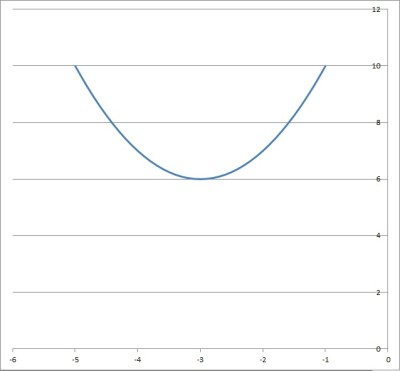Here, we have the parabola y = x2 + 6x + 15, with a vertex at the point (-3, 6).

According to our equation from earlier, the focus is the point (h, k + 1/4a).

Substituting a = 1, h = -3, and k = 6, we get:

• an x-coordinate of h = -3
• a y-coordinate of k + 1/4a = 6 + 1/4(1) = 6 + 1/4 = 6.25

So, the focus of this parabola is the point (-3, 6.25).

Likewise, the directrix is the line y = k – (1/4a), which is y = 5.75 when a = 1.

You can see the focus and directrix illustrated on the graph below.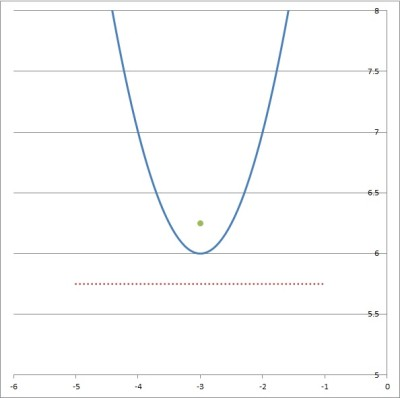Here, we have the parabola y = (x+3)2 +6 (blue curve), along with the focus at the green point (-3, 6.25) and the directrix y = 5.75 (horizontal red line).

#### Example 2: Find The Focus Of A Parabola In Standard Form

Let’s say we have the following quadratic equation in standard form:

• y = -2x2 + 3x – 4

In this case, a = -2, b = 3 and c = -4. You can see the graph below.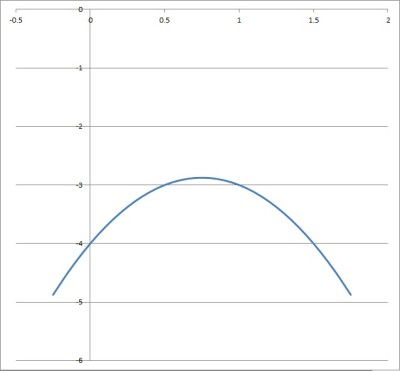Here, we have the parabola y = -2x2 + 3x – 4, with a vertex at the point (3/4, -23/8).

Let’s use the formula for the focus: (-b/2a, (4ac – b2 + 1)/4a) as a shortcut.

To find the x-coordinate:

• x = -b/2a
• x = -3/2(-2)
• x = -3/-4
• x = ¾
• x = 0.75

To find the y-coordinate:

• y = (4ac – b2 + 1)/4a
• y = (4(-2)(-4) – 32 + 1)/4(-2)
• y = (32 – 9 + 1)/-8
• y = (24)/-8
• y = -3

So, the focus of the parabola is the point (0.75, -3).

Likewise, the directrix is the line y = (4ac – b2 – 1)/4a  which is:

• y = (4ac – b2 – 1)/4a
• y = (4(-2)(-4) – 32 – 1)/4(-2)
• y = (32 – 9 – 1)/-8
• y = (22)/-8
• y = -11/4
• y = -2.75

You can see the focus and directrix illustrated on the graph below.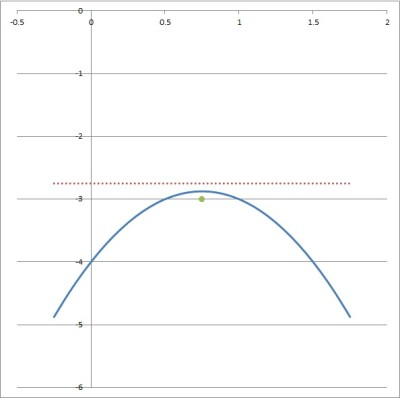Here, we have the parabola y = -2(x-0.75)2 – 2.875 (blue curve), along with the focus at the green point (0.75, -3) and the directrix y = -2.75 (horizontal red line).

### How To Find The Focus Of A Parabola On A Graph

To find the focus of a parabola on a graph, we can use one of the methods above:

• Find the vertex (h, k) and the quadratic coefficient a from the graph and use the formula (h, k + 1/4a).
• Find the standard form y = ax2 + bx + c from the graph and use the shortcut formula (-b/2a, (4ac – b2 + 1)/4a).

Let’s look at an example.

#### Example: Find The Focus Of A Parabola On A Graph

Let’s say we have the graph of a parabola given below.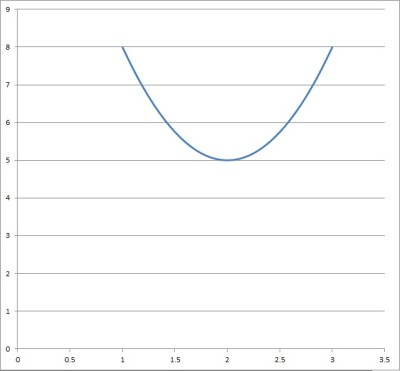Here, we have the parabola y = 3(x-2)2 + 5, with a vertex at the point (2, 5).

As you can see, we know the vertex (2, 5) and the point (0, 17) are on the graph, but that is enough to find what we need: h, k, and a.

Since we know the vertex is (2, 5) = (h, k), then h = 2 and k = 5.  This means that the vertex form of our parabola looks like this:

• y = a(x – h)2 + k
• y = a(x – 2)2 + 5

Substituting the point (x, y) = (0, 17), we get:

• y = a(x – 2)2 + 5
• 17 = a(0 – 2)2 + 5
• 17 = a(–2)2 + 5
• 17 = a(4)+ 5
• 12 = a(4)
• 3 = a

So, our values are a = 3, h = 2, and k = 5.  Then we use our formula for the focus:

• (h, k + 1/4a)
• (2, 5 + 1/4(3))
• (2, 5 + 1/12)
• (2, 61/12)

So the focus of the parabola is the point (2, 61/12).  Likewise, the directrix is y = k – 1/4a = 5 – 1/4(3) = 5 – 1/12 = 59/12.

You can see the focus and directrix illustrated on the graph below.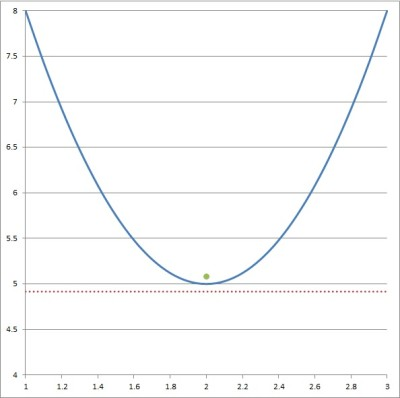Here, we have the parabola y = 3(x-2)2 + 5 (blue curve), along with the focus at the green point (2, 61/12) and the directrix y = 59/12 (horizontal red line).

## Conclusion

Now you know what the focus of a parabola is, along with how it is related to the directrix and the equation of a parabola.

You can learn how to find the vertex of a parabola with 3 methods in this article.

You can learn how to find the domain and range of a parabola here.

You can learn about the related concept of hyperbola (and how to graph them) here.